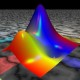Ask Your Question

# Unable to simplify variable completely

In solving the following hicksian demands in this problem and so far the proceedure works great.

x1, x2, l, p1, p2, a, b,  R= var('x1, x2, l, p1, p2, a, b,  R')
U = x1^a*x2^b
m = p1*x1+p2*x2;
L = m+ l * (R-U);
dLdx = L.diff(x1);
dLdy = L.diff(x2);
dLdl = L.diff(l);
step1=solve([dLdx == 0, dLdy == 0, dLdl == 0], p1, p2, R)
step2=solve((p1/p2).subs(step1)==p1/p2, x1)
good2step3 = solve((U/R).subs(step2).log().log_expand()==0, x2)
good1step4=solve(step2.subs(good2step3).canonicalize_radical(),x1)


however I note that I dont get such a clean result for good2step3, though algebraically correct its not completely simplified. I've tried simplify_rational() and simplify_log but that ends up ruining my clean solution for x1 in good1step4.

Is there more I can do to clean up my solutions?

edit retag close merge delete

## 1 Answer

Sort by » oldest newest most votedDon't expect a clean expression from the output of solve. Instead, print (or show) the solution by substitution and apply additional methods to simplify the expressions. In this case, you can replace the last line by

good1step4 = solve(step2.subs(good2step3),x1)
print("x2 =", x2.subs(good2step3).canonicalize_radical())
print("x1 =", x1.subs(good1step4).canonicalize_radical())

more

## Your Answer

Please start posting anonymously - your entry will be published after you log in or create a new account.

Add Answer

## Stats

Asked: 2020-07-17 22:04:37 +0200

Seen: 65 times

Last updated: Jul 20 '20